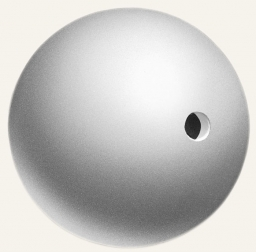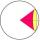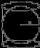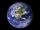The radius of the sphere we reduce by 1/3 of the original radius. How much percent does the volume and surface of the sphere change?

p1 =  70.3704 %
p2 =  55.5556 %

### Step-by-step explanation:Did you find an error or inaccuracy? Feel free to write us. Thank you!Tips to related online calculators
Need help to calculate sum, simplify or multiply fractions? Try our fraction calculator.
Check out our ratio calculator.
Tip: Our volume units converter will help you with the conversion of volume units.

#### You need to know the following knowledge to solve this word math problem:

We encourage you to watch this tutorial video on this math problem:

## Related math problems and questions:How many times does the surface of a sphere decrease if we reduce its radius twice?
• The ballThe ball has a radius of 2m. What percentage of the surface and volume is another sphere whose radius is 20% larger?
• Sphere vs cubeHow many % of the surface of a sphere of radius 12 cm is the surface of a cube inscribed in this sphere?
• SphereIntersect between plane and a sphere is a circle with a radius of 60 mm. Cone whose base is this circle and whose apex is at the center of the sphere has a height of 34 mm. Calculate the surface area and volume of a sphere.
• Inscribed sphereHow many % of the volume of the cube whose edge is 6 meters long is a volume of a sphere inscribed in that cube?
• Surface area 2Calculate how many % reduce the surface area of the cube is we reduced length of each edge by 10%.
• Spherical capFrom the sphere of radius 11 was truncated spherical cap. Its height is 6. What part of the volume is a spherical cap from the whole sphere?
• Cube in sphereThe cube is inscribed in a sphere with a radius r = 6 cm. What percentage is the volume of the cube from the volume of the ball?
• Reduce of the volumeCalculate how many % reduce the volume of the cube is we reduced length of each edge by 10%.
• Cylinder surface, volumeThe area of the cylinder surface and the cylinder jacket are in the ratio 3: 5. The height of the cylinder is 5 cm shorter than the radius of the base. Calculate surface area and volume of the cylinder.
• Cube, cuboid, and sphereVolumes of a cube and a cuboid are in ratio 3: 2. Volumes of sphere and cuboid are in ratio 1: 3. At what rate are the volumes of cube, cuboid, and sphere?
• Cube zoomHow many percents do we increase the volume and surface of the cube if we magnify its edge by 38 %?
• Sphere cutA sphere segment is cut off from a sphere k with radius r = 1. The volume of the sphere inscribed in this segment is equal to 1/6 of the segment's volume. What is the distance of the cutting plane from the center of the sphere?
• Percent changeIf the length of a rectangle is increased by 25% and the width is decreased by 10%, the area of the rectangle is larger than the area of the original rectangle by what percent?
• Equilateral cylinderA sphere is inserted into the rotating equilateral cylinder (touching the bases and the shell). Prove that the cylinder has both a volume and a surface half larger than an inscribed sphere.
• Lateral surface areaThe ratio of the area of the base of the rotary cone to its lateral surface area is 3: 5. Calculate the surface and volume of the cone, if its height v = 4 cm.
• Earth's surfaceThe greater part of the earth's surface (r = 6371 km) is covered by oceans; their area is approximately 71% of the Earth's surface. What is the approximate area of the land?